# Graphing electric potential for two positive charges

• ChiralSuperfields
In summary, the conversation revolved around a graphing problem where the speaker had to plot the electric potential as units of ## \frac{k_eQ}{a} ## in comparison to a textbook plot. The speaker initially drew the graph freehand, but was advised to use a plotting program for accuracy. After some back and forth, the speaker shared a new graph that was corrected in terms of the x-axis, but still had incorrect labels on the y-axis. The speaker also had some confusion about the notation used for the x-axis and asked for clarification.

#### ChiralSuperfields

Homework Statement
Relevant Equations
For part (a) of this problem,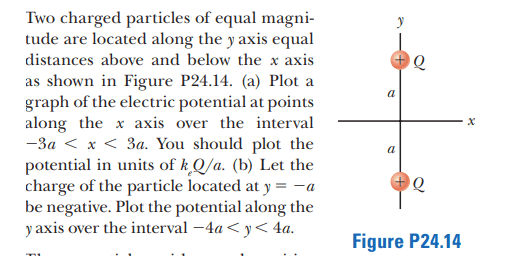The solution is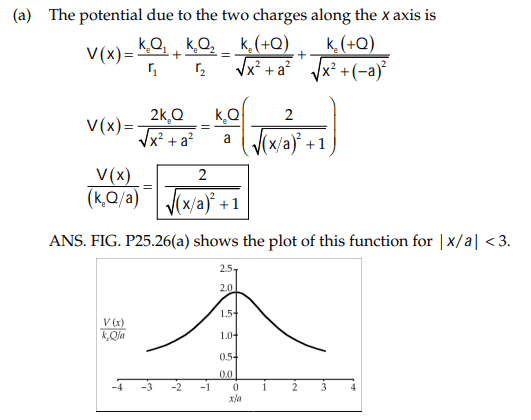However, my solution is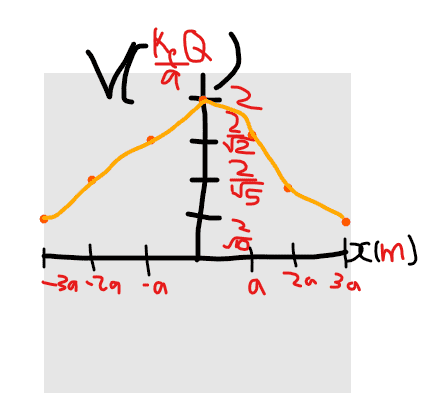Am I correct? In the solutions that don't appear to plot the electric potential as units of ## \frac {k_eQ} {a} ## like I have which the problem statement said to do.

Many thanks!

Your plot is too crudely drawn for a direct comparison with the textbook. Not only that, but in your plot the tick marks on the vertical axis are not equally spaced as is the case in the textbook plot. So what exactly are you comparing? I suggest that you use a plotting program instead of doing it freehand. Free plotting programs exist on the internet. I went to https://www.desmos.com/calculator and created this.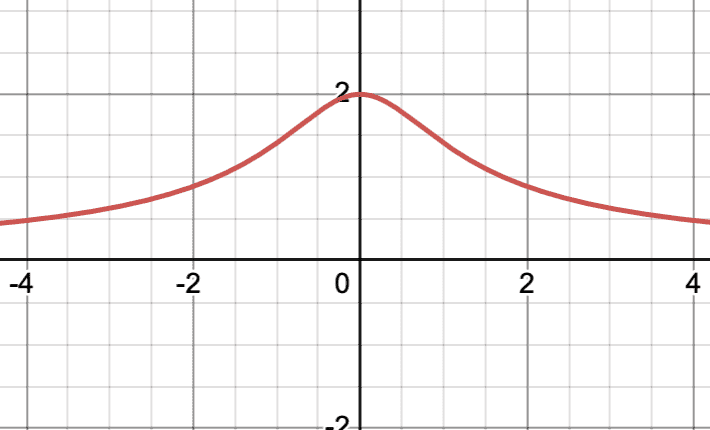•PhDeezNutz and ChiralSuperfields
kuruman said:
Your plot is too crudely drawn for a direct comparison with the textbook. Not only that, but in your plot the tick marks on the vertical axis are not equally spaced as is the case in the textbook plot. So what exactly are you comparing? I suggest that you use a plotting program instead of doing it freehand. Free plotting programs exist on the internet. I went to https://www.desmos.com/calculator and created this.

View attachment 321043
Yes sorry @kuruman I will graph it. I am comparing my graph which has a y-axis of ## V(\frac{k_eQ}{a}) = \frac {2}{\sqrt{10}}, \frac {2}{\sqrt{5}}, \frac {2}{\sqrt{2}}, 2## and x-axis of## x(a) = -3a, -2a, -a, a, 2a, 3a ##

Last edited:
kuruman said:
Your plot is too crudely drawn for a direct comparison with the textbook. Not only that, but in your plot the tick marks on the vertical axis are not equally spaced as is the case in the textbook plot. So what exactly are you comparing? I suggest that you use a plotting program instead of doing it freehand. Free plotting programs exist on the internet. I went to https://www.desmos.com/calculator and created this.

View attachment 321043
Here is my new graph @kuruman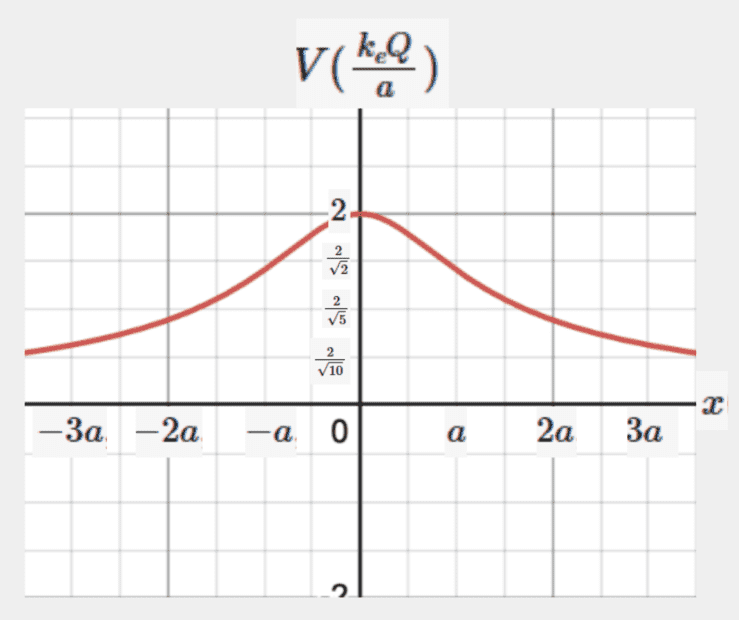I am comparing the y and x axes. I am not sure whether my graph is correct or not.

Thanks!

#### Attachments

It is not correct. The x-axis is fine and compares directly with the textbook and my plot if ##a=1##, i.e. plot ##k_eQ/ a## vs. ##x/a##. The y-axis is not OK. You cannot label equally spaced intervals using unequal labels. Since there are four intervals between zero and 2 on the vertical axis, the labels should be 0.5, 1.0 and 1.5. In fact the demos plotting utility assumes that this is the case.

•ChiralSuperfields
kuruman said:
It is not correct. The x-axis is fine and compares directly with the textbook and my plot if ##a=1##, i.e. plot ##k_eQ/ a## vs. ##x/a##. The y-axis is not OK. You cannot label equally spaced intervals using unequal labels. Since there are four intervals between zero and 2 on the vertical axis, the labels should be 0.5, 1.0 and 1.5. In fact the demos plotting utility assumes that this is the case.
Thank you for your reply @kuruman ! Whoops I see my mistake with the y-axis. Also, I've never seen the notation ##x/a## - what does it mean please?

I will post a new graph soon.

kuruman said:
It is not correct. The x-axis is fine and compares directly with the textbook and my plot if ##a=1##, i.e. plot ##k_eQ/ a## vs. ##x/a##. The y-axis is not OK. You cannot label equally spaced intervals using unequal labels. Since there are four intervals between zero and 2 on the vertical axis, the labels should be 0.5, 1.0 and 1.5. In fact the demos plotting utility assumes that this is the case.
Here's the new graph @kuruman ,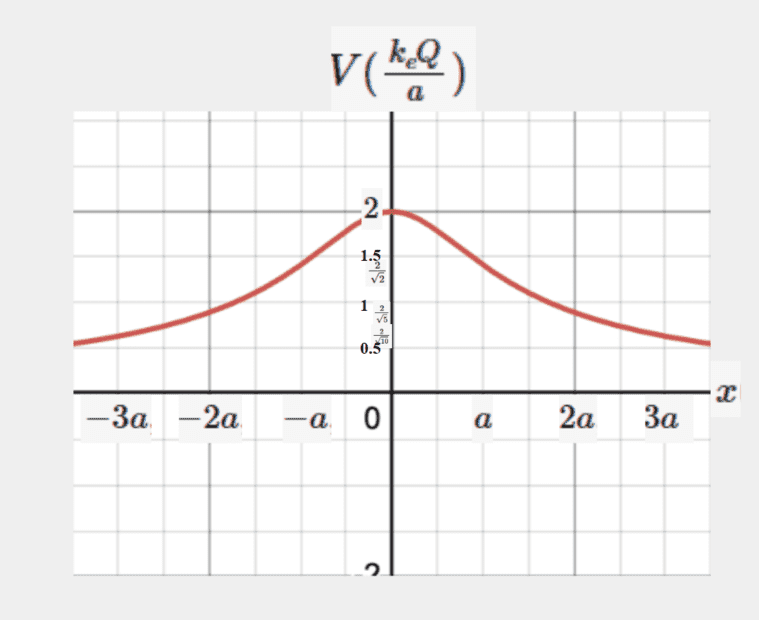That's fine except for the old labels on the vertical axis that need to be removed. Just so that you know. You don't have to do it because it's not going to be graded.

•ChiralSuperfields
kuruman said:
That's fine except for the old labels on the vertical axis that need to be removed. Just so that you know. You don't have to do it because it's not going to be graded.
Thank you @kuruman , but I still don't see how my and their graphs are equivalent. On their graph the y-axis is ## \frac {V(x)a} {k_eQ}## and my graph it is ## V (\frac {k_eQ} {a}) ##

For the x-axis, their graph is ## x/a ## and my graph is ## x ##.

Correct me if I am wrong, but the notation ## x ## is the same as ## x(a) ##. I'm not sure why.

Many thanks!

Callumnc1 said:
Thank you @kuruman , but I still don't see how my and their graphs are equivalent. On their graph the y-axis is ## \frac {V(x)a} {k_eQ}## and my graph it is ## V (\frac {k_eQ} {a}) ##

For the x-axis, their graph is ## x/a ## and my graph is ## x ##.

Correct me if I am wrong, but the notation ## x ## is the same as ## x(a) ##. I'm not sure why.

Many thanks!
EDIT: Sorry I meant that the notation ## x ## is not the same as ## x(a) ##

This may help https://www.desmos.com/calculator/zu8hbssbxp . (Visit to see how the pieces below are created.)

Vary the a-slider.

(By the way, you can copy-paste equations from desmos (as TeX, acceptable for MathJax)... just surround by itex and /itex in square brackets .)

$V_{factor}=\frac{2}{\sqrt{\left(x_{factor}\right)^{2}+1}}$ (right-click the equation)
where
$V=\frac{kQ}{a}\left(\frac{2}{\sqrt{\left(\frac{x}{a}\right)^{2}+1}}\right)$

$V_{fac}=\frac{V}{\left(\frac{kQ}{a}\right)}$ and $x_{fac}=\frac{x}{a}$ (different variable names given in Desmos) are both dimensionless quantities.
($\frac{kQ}{a}$ carries the units of Volts, and $\frac{x}{a}$ carries the units of meters.)

Note that if the [implied] right-hand-side of an entry has a free variable, it becomes the independent variable.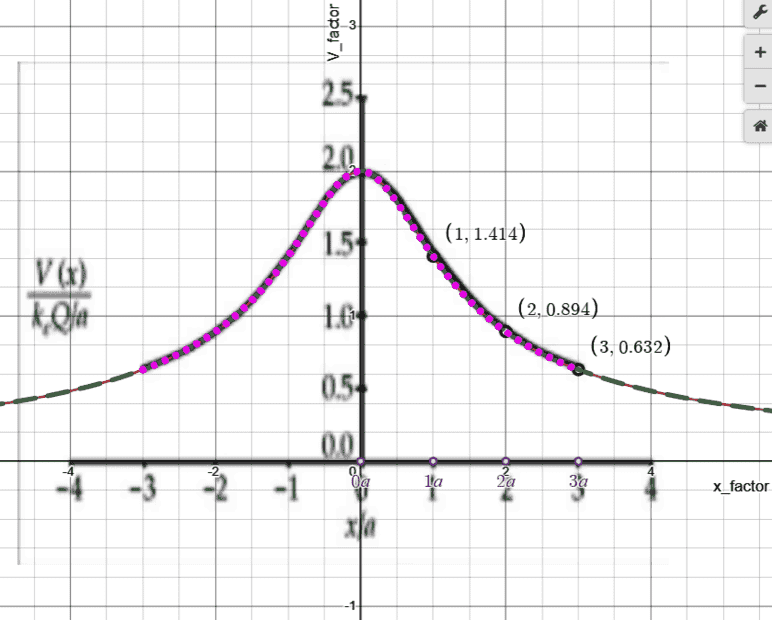Last edited:
•ChiralSuperfields and kuruman
robphy said:
This may help https://www.desmos.com/calculator/zu8hbssbxp . (Visit to see how the pieces below are created.)

Vary the a-slider.

(By the way, you can copy-paste equations from desmos (as TeX, acceptable for MathJax)... just surround by itex and /itex in square brackets .)

$V_{factor}=\frac{2}{\sqrt{\left(x_{factor}\right)^{2}+1}}$ (right-click the equation)
where
$V=\frac{kQ}{a}\left(\frac{2}{\sqrt{\left(\frac{x}{a}\right)^{2}+1}}\right)$

$V_{fac}=\frac{V}{\left(\frac{kQ}{a}\right)}$ and $x_{fac}=\frac{x}{a}$ (different variable names given in Desmos) are both dimensionless quantities.
($\frac{kQ}{a}$ carries the units of Volts, and $\frac{x}{a}$ carries the units of meters.)

Note that if the [implied] right-hand-side of an entry has a free variable, it becomes the independent variable.

View attachment 321094
Thank you for your reply @robphy ! I kind of get what you are saying that my and their graphs are equivalent, is that correct?

Many thanks!

Type in your equation in that Desmos file and see if it matches.

•ChiralSuperfields
robphy said:
Type in your equation in that Desmos file and see if it matches.
Thank you for your reply @robphy! How do I do that sorry?

Many thanks!

On that Desmos file, add a blank entry or scroll down to find one blank entry.

•ChiralSuperfields
robphy said:
On that Desmos file, add a blank entry or scroll down to find one blank entry.